# How to Build a Tic Tac Toe Game in Python

Learn how to build a tic tac toe game using the Pygame library from scratch in Python.
21 min read · Updated apr 2023 · Game Development

Confused by complex code? Let our AI-powered Code Explainer demystify it for you. Try it out!

Tic-Tac-Toe is a two-player game in which the main goal is to be the first one to draw a linear pattern that consists of three of your selected character (either `"X"` for the first player and `"O"` for the second player) in a 3x3 table.

The winning pattern can be horizontal, vertical, or diagonal, as long as the characters are aligned. This tutorial covers how you create a Tic-Tac-Toe game using Python with the Pygame module, and I will guide you from setting up to creating the game methods, up to styling the game interface.

## Installation and Setup

Let's start by creating first a Python file and name it `tictactoe.py`. After that, let's add the imports for this project if you haven't installed the `Pygame` module yet, you can do `pip install pygame` for Windows or `pip3 install pygame` for Linux and Mac.

``````import pygame
from pygame.locals import *

pygame.init()
pygame.font.init()``````

The `.init()` functions we call start the game environment, allowing us to use Pygame modules instead of importing all of them manually. Then, in the same directory as `tictactoe.py`, create a directory called `images`, this directory serves as the container of the images we're using later on. Inside the `images` directory, let's paste our two pictures; the `Tc-O.png` for the `"O"` character and the `Tc-X.png` for the `"X"` character.Before we proceed let's try first if this is working. We'll do a simple `Hello World` in the game window:

``````pygame.init()
pygame.font.init()

font = pygame.font.SysFont("Courier New", 40)

running = True

screen = pygame.display.set_mode((450, 500))
screen.fill("brown")

message = font.render("Hello World!!", True, ("blue"))
screen.blit(message,(0,0))

pygame.display.update()

while running:
for event in pygame.event.get():
if event.type == pygame.QUIT:
running = False
pygame.quit()``````

After the game runs on the terminal, the game window will pop up on the screen with the message "Hello World" at the corner: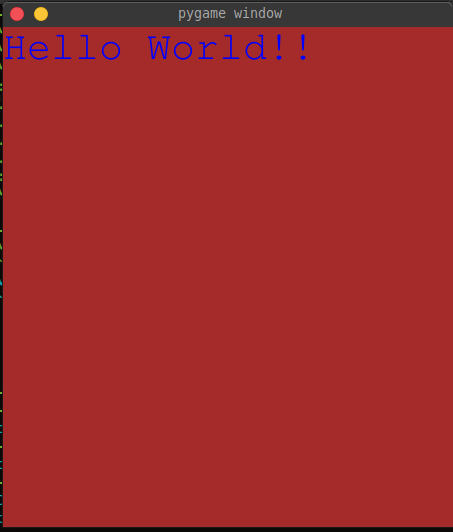Now that we see it's all working, we can delete these lines except the imports and initializers and we are good to hop on the next step.

Let's start over by adding our window size (length, height) and creating the window with the caption "Tic Tac Toe":

``````window_size = (450, 500)
cell_size = 150

screen = pygame.display.set_mode(window_size)
pygame.display.set_caption("Tic Tac Toe")``````

## The Tic-Tac-Toe Class

Now create the `TicTacToe` class. The class is supposed to have an `__init__()` method which initializes various instance variables, sets up the game board, and sets the colors and fonts used in the game:

``````class TicTacToe():

def __init__(self, table_size):
self.table_size = table_size
self.cell_size = table_size // 3
self.table_space = 20
self.table = []
for col in range(3):
self.table.append([])
for row in range(3):
self.table[col].append("-")

self.player = "X"
self.winner = None
self.taking_move = True
self.running = True

self.background_color = (255, 174, 66)
self.table_color = (50, 50, 50)
self.line_color = (190, 0, 10)
self.instructions_color = (17, 53, 165)
self.game_over_bg_color = (47, 98, 162)
self.game_over_color = (255, 179, 1)
self.font = pygame.font.SysFont("Courier New", 35)
self.FPS = pygame.time.Clock()``````

The `self.table_size` parameter is used to determine the size of the game board (the value is for the table's length and height). The `self.cell_size` variable is set to the size of each cell on the board, calculated as `table_size // 3` since we're using a 3x3 table.

The `self.player` is set to `"X"` initially and the `self.winner` is set to `None` as the default value. The `self.taking_move` remains `True` as long as there is no winner or the game isn't over yet, and the `self.running` will remain `True` until the user hit the exit icon on the game. These variables are used to keep track of the state of the game.

The `self.table` is a 2D list representing the game board. The outer loop runs three times, iterating over the three columns on the game board. For each column, a new empty list is appended to the table list, creating a 2D array of 3 columns. The inner loop then runs three times for each column, iterating over the three rows on the game board. For each row, a new empty cell containing `"-"` is appended to the current column list, creating a 2D array of 3 rows and 3 columns.Below are the variables set to different RGB color values, which are used to color various parts of the game. The `font` variable is set to a `SysFont` object, which is used to render text in the game. The `FPS` variable is a Pygame clock object, used to regulate the frame rate of the game.

## Calling the Game

Let's create a new function and name it `main()`, this method will be responsible for updating the Pygame display, processing user input events, and regulating the frame rate of the game:

``````    def main(self):
screen.fill(self.background_color)

while self.running:
for self.event in pygame.event.get():
if self.event.type == pygame.QUIT:
self.running = False

pygame.display.flip()
self.FPS.tick(60)``````

Within the `while` loop, the method listens for Pygame events, such as mouse clicks or keyboard presses, using the `pygame.event.get()`. If a `QUIT` event is detected, such as the player clicking the `"X"` button to close the game window, the `self.running` variable is set to `False`, causing the game loop to terminate and the game to exit. After checking for events, the method calls `pygame.display.flip()`, which updates the contents of the Pygame display surface to the screen. We'll update this function later on as we grow our code but for now, we can simply try to run our game to see the changes.

At the lower-most, outside of the class, let's instantiate the `TicTacToe` class:

``````if __name__ == "__main__":
g = TicTacToe(window_size)
g.main()``````

## Drawing the Table

Now that we have a nice window game with a good background color, we can start creating the table representation for our game.

As you remember earlier, we coded above the `self.table` which serves as the main table where we can put player characters in empty cells (has a value of `"-"` while they are empty). This table we're drawing is for representation of that table where we add lines that represent the rows, columns, and cells of the table. Below the `__init__()`, create a new function called `_draw_table()`:

``````    # draws table representation
def _draw_table(self):
tb_space_point = (self.table_space, self.table_size - self.table_space)
cell_space_point = (self.cell_size, self.cell_size * 2)
r1 = pygame.draw.line(screen, self.table_color, [tb_space_point, cell_space_point], [tb_space_point, cell_space_point], 8)
c1 = pygame.draw.line(screen, self.table_color, [cell_space_point, tb_space_point], [cell_space_point, tb_space_point], 8)
r2 = pygame.draw.line(screen, self.table_color, [tb_space_point, cell_space_point], [tb_space_point, cell_space_point], 8)
c2 = pygame.draw.line(screen, self.table_color, [cell_space_point, tb_space_point], [cell_space_point, tb_space_point], 8)``````

Here we draw the table that can be responsive to the size of the `self.table_size`. The `tb_space_point` contains a tuple that has two values of spacing, which can be x or y in drawing the lines.

We don't want our table rows and columns to be hitting the sides of the window so we put a space value of 20 per side. The `tb_space_point` is responsible for giving spaces for the left and top of the table while the `tb_space_point` is responsible for the right and the bottom side.

Same as the `tb_space_point`, the `cell_space_point` is also for giving x and y points. But unlike `tb_space_point`, its focus is to identify the height and length of the cell, giving each part of the cell an equal value of spaces. The `cell_space_point` serves as the y-axis in both points of the first row (`r1`), and the x-axis at starting point of both column lines (`c1`, `c2`). On the other hand the `cell_space_point` serves as the y-axis in both points of the 2nd row (`r2`), and the x-axis for in ending point of both column lines(`c1`, `c2`).

We gave each line a weight of 8 which fits it better. Now back to the `main()`, let's call the `_draw_table()` before the `while` loop, below the screen fill:

``````    def main(self):
screen.fill(self.background_color)
self._draw_table()

while self.running:
for self.event in pygame.event.get():
...``````

Let's see our table drawing by running the game.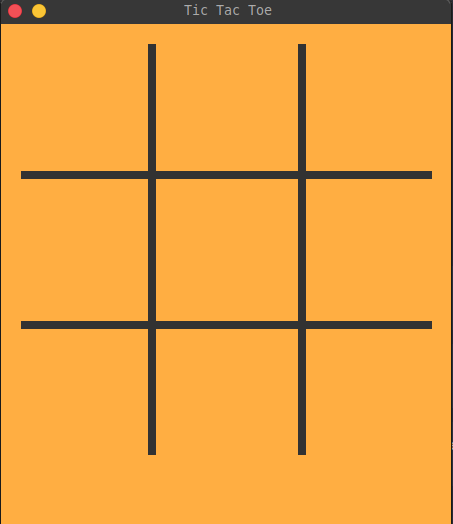Note: we keep this game window `450x500` so we can use the 450 height and 450 length for the table, and the remaining space of 50 below for game instructions/messages.

## Making a Move

That's a nice table we have right there. Now let's create a function for adding a move and changing the player. Below `_draw_table()`, create `_change_player()` function and `_move()` function:

``````    def _change_player(self):
self.player = "O" if self.player == "X" else "X"``````

The `_change_player()` method simply switches the current player between `"X"` and `"O"`. If the current player is `"X"`, the method assigns the `"O"` value to the `self.player` variable, and vice versa.

``````    # processing clicks to move
def _move(self, pos):
try:
x, y = pos // self.cell_size, pos // self.cell_size
if self.table[x][y] == "-":
self.table[x][y] = self.player
self._draw_char(x,y,self.player)
self._game_check()
self._change_player()
except:
print("Click inside the table only")``````

The `_move()` method handles user clicks on the game board. It takes a `pos` argument that represents the position of the user's click on the game board.

The method first calculates the corresponding row (`x`) and column (`y`) of the game board where the user has clicked by dividing the coordinates of the click by the size of each cell on the board (`self.cell_size`).

It then checks if the cell at the clicked position on the game board is empty (represented by the `"-"` string in the `self.table` list). If it is empty, the method updates the `self.table` list to place the current player's symbol (`"X"` or `"O"`) in the clicked cell.

The method then calls `_draw_char()`, which draws the player's symbol on the game board in the correct cell. After that, it calls `_game_check()`, for checking if the game is over or not (if there is a winner or a tie).

Finally, the method calls `_change_player()`, which switches the current player to the other player so that the other player can take their turn. If the user clicks outside of the game board or an error occurs, the method prints a message to the console indicating that the user should "click inside the table only".

Since we currently don't have the `_draw_char()` and the `_game_check()`, you can simply comment them out for a while if you want to try running the game to prevent catching errors and uncomment them once we have those functions. Now let's update our `main()` and add catching mouse clicks by recognizing the event `MOUSEBUTTONDOWN`:

``````    def main(self):
screen.fill(self.background_color)
self._draw_table()
while self.running:
# self._message()
for self.event in pygame.event.get():
if self.event.type == pygame.QUIT:
self.running = False

if self.event.type == pygame.MOUSEBUTTONDOWN:
if self.taking_move:
self._move(self.event.pos)

pygame.display.flip()
self.FPS.tick(60)``````

We comment out the `_message()` method as well since we currently don't have it but we'll create it later.

As we discussed earlier, The `_draw_char()` method draws the current player's symbol (either `"X"` or `"O"`) on the game board in the selected cell. The method takes three arguments; x and y, which represents the row and column of the selected cell, and `player`, which represents the current player (`"X"` or `"O"`). Below the `_move()` function, create `_draw_char()` function:

``````    # draws character of the recent player to the selected table cell
def _draw_char(self, x, y, player):
if self.player == "O":
elif self.player == "X":
img = pygame.transform.scale(img, (self.cell_size, self.cell_size))
screen.blit(img, (x * self.cell_size, y * self.cell_size, self.cell_size, self.cell_size))``````

First, the method checks whether the `player` variable is equal to `"O"` or `"X"`. Based on the value of a player, the method loads the corresponding image file (`Tc-O.png` or `Tc-X.png`) using `pygame.image.load()`. The image file is then scaled down to the size of a single game cell using the `pygame.transform.scale()`, with the dimensions of the scaled image set to `self.cell_size`.

Finally, the image is blitted (drawn) onto the game board using `screen.blit()`, with the position of the blit determined by the `(x * self.cell_size, y * self.cell_size)` coordinates, and the size of the blit is determined by `(self.cell_size, self.cell_size)` tuple to set the height and length.

We can now uncomment the line `self._draw_char(x,y,self.player)` written in the `_move()` method. Let's try to run it if it works.

Let's try to click a random cell first:And let's try another blank cell to see if it really changes the player: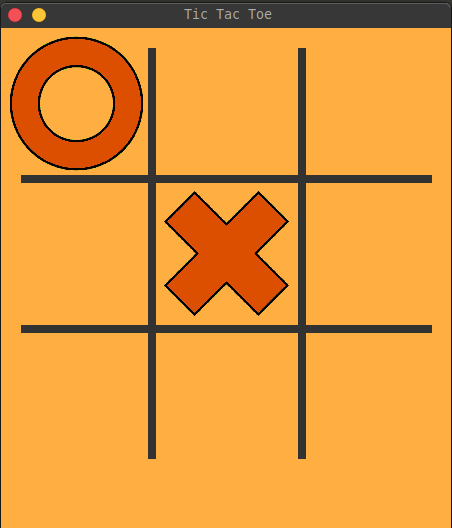And if we click outside of the table but still inside of the window, we can see this message on the terminal (I clicked outside multiple times).## Creating Game Messages

Before adding the function for checking, let's add first the `_message()` function so it is easier to figure out what's happening in the game. The `_message(``)` method displays messages on the screen indicating the current game state, such as which player's turn it is, who has won the game, or if the game ended in a draw:

``````    # instructions and game-state messages
def _message(self):
if self.winner is not None:
screen.fill(self.game_over_bg_color, (130, 445, 193, 35))
msg = self.font.render(f'{self.winner} WINS!!', True, self.game_over_color)
screen.blit(msg,(144,445))
elif not self.taking_move:
screen.fill(self.game_over_bg_color, (130, 445, 193, 35))
instructions = self.font.render('DRAW!!', True, self.game_over_color)
screen.blit(instructions,(165,445))
else:
screen.fill(self.background_color, (135, 445, 188, 35))
instructions = self.font.render(f'{self.player} to move', True, self.instructions_color)
screen.blit(instructions,(135,445))``````

If `self.winner` is not `None`, then the game is over and the message displayed on the screen says who won the game. In this case, the method first fills a rectangular area on the screen with the `self.game_over_bg_color` color using the `screen.fill()` method, with the `(130, 445, 193, 35)` coordinates indicating the location and size of the rectangular area.

The method then renders the message "X/O WINS!!" using the `self.font.render()` method, with the winner variable indicating which player won the game. The message is rendered in the `game_over_color` color using the `True` argument and the message is blitted onto the screen using the `screen.blit()`, with the `(144,445)` coordinates indicating the location of the message on the screen.

If `self.winner` is `None` and `self.taking_move` is `False`, then the game has ended in a draw, and the method displays the message "DRAW!!" on the screen using a similar approach as above, but with the message and coordinates adjusted accordingly.

Otherwise, the game is still ongoing and the method displays a message indicating which player's turn it is. The method fills a rectangular area on the screen with the `self.background_color` color and then renders the message "X/O to move" using the `self.font.render()` method, with the player variable indicating whose turn it is. The message is rendered in the `self.instructions_color` color, and then blitted onto the screen using the `screen.blit()`, with the `(135,445)` coordinates indicating the location of the message on the screen.

Let's try to run it again if it really shows messages.

Instructions for the player "X".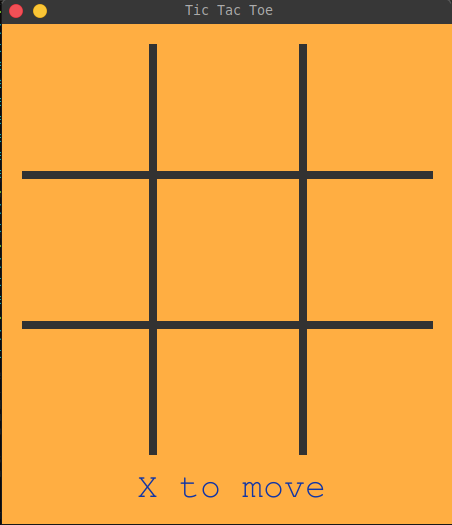Instructions for the player "O".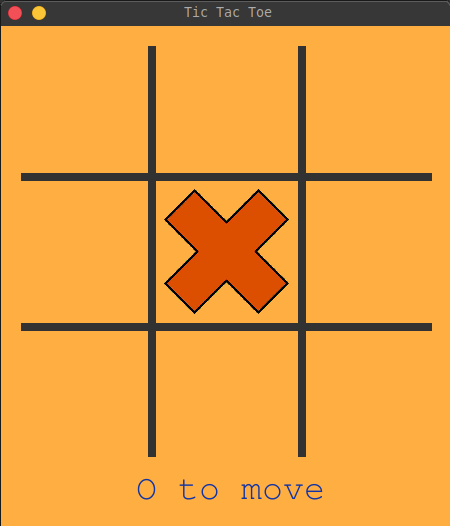## Checking the Table: Looking for a Winner

For checking the board, create the `_game_check()` below the `_message()` function. In this one, we're going to create five different `for` loops for checking vertically (by column), horizontally (by row), diagonally (one for left-top to right-bottom and one for right-top to left-bottom), and another one for checking blank cells:

``````    def _game_check(self):
# vertical check
for x_index, col in enumerate(self.table):
win = True
pattern_list = []
for y_index, content in enumerate(col):
if content != self.player:
win = False
break
else:
pattern_list.append((x_index, y_index))
if win == True:
self._pattern_strike(pattern_list,pattern_list[-1],"ver")
self.winner = self.player
self.taking_move = False
self._message()
break

# horizontal check
for row in range(len(self.table)):
win = True
pattern_list = []
for col in range(len(self.table)):
if self.table[col][row] != self.player:
win = False
break
else:
pattern_list.append((col, row))
if win == True:
self._pattern_strike(pattern_list,pattern_list[-1],"hor")
self.winner = self.player
self.taking_move = False
self._message()
break

# left diagonal check
for index, row in enumerate(self.table):
win = True
if row[index] != self.player:
win = False
break
if win == True:
self._pattern_strike((0,0),(2,2),"left-diag")
self.winner = self.player
self.taking_move = False
self._message()

# right diagonal check
for index, row in enumerate(self.table[::-1]):
win = True
if row[index] != self.player:
win = False
break
if win == True:
self._pattern_strike((2,0),(0,2),"right-diag")
self.winner = self.player
self.taking_move = False
self._message()

# blank table cells check
blank_cells = 0
for row in self.table:
for cell in row:
if cell == "-":
blank_cells += 1
if blank_cells == 0:
self.taking_move = False
self._message()``````

For vertical check, it goes through each column in the table and checks if all the cells in that column are occupied by the same player. If yes, it calls the `_pattern_strike()` method to highlight the winning pattern and sets the winner attribute to the current player. It also sets `self.taking_move` to False and calls the `_message()` method to display the winner.

The horizontal check is similar to the vertical check, but it goes through each row instead of columns.

The left diagonal check starts at the top-left cell and checks if all the cells in the diagonal direction are occupied by the same player. If yes, it calls the `_pattern_strike()` method to highlight the winning pattern and sets the winner attribute to the current player. It also sets `self.taking_move` to False and calls the `_message()` method to display the winner.

The right diagonal check is similar to the left diagonal check, but it starts at the top-right cell and goes towards the bottom-left cell.

Finally, if all cells in the table are occupied and there is no winner yet, the method sets `self.taking_move` to `False` and calls the `_message()` method to display the draw message.

Now we can also uncomment the line `self._game_check()` in the `_move()` function. If we try to run the game instantly in this stage, we'll get an outcome of getting the message "Click inside the table only" at the terminal once we hit the winning patterns.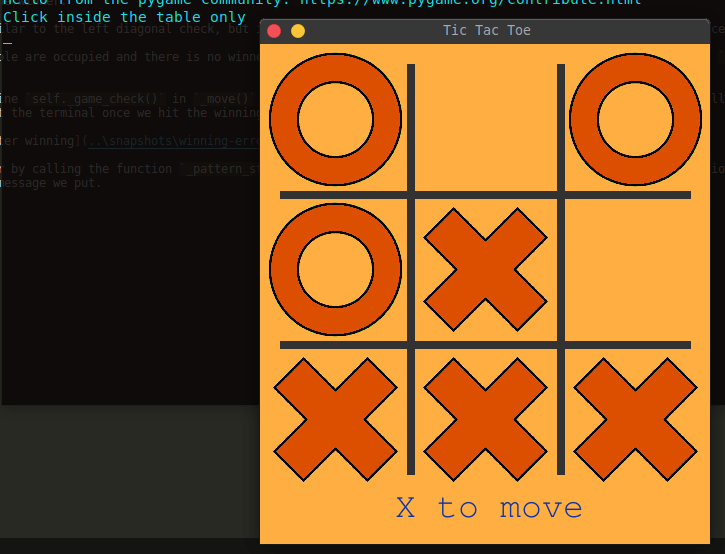It is because of having an error by calling the function `_pattern_strike()` once we have a winner and since we don't have that function, that causes the error and cached by except, making it print the message we put.

## Striking Winning Pattern

And now, let's finish this game. Below the `_game_check()`, create a method and name it `_pattern_strike()`. This function is responsible for drawing a line to represent the winning pattern if a player has won the game:

``````    # strikes a line to winning patterns if already has
def _pattern_strike(self, start_point, end_point, line_type):
# gets the middle value of the cell
mid_val = self.cell_size // 2

# for the vertical winning pattern
if line_type == "ver":
start_x, start_y = start_point * self.cell_size + mid_val, self.table_space
end_x, end_y = end_point * self.cell_size + mid_val, self.table_size - self.table_space

# for the horizontal winning pattern
elif line_type == "hor":
start_x, start_y = self.table_space, start_point[-1] * self.cell_size + mid_val
end_x, end_y = self.table_size - self.table_space, end_point[-1] * self.cell_size + mid_val

# for the diagonal winning pattern from top-left to bottom right
elif line_type == "left-diag":
start_x, start_y = self.table_space, self.table_space
end_x, end_y = self.table_size - self.table_space, self.table_size - self.table_space

# for the diagonal winning pattern from top-right to bottom-left
elif line_type == "right-diag":
start_x, start_y = self.table_size - self.table_space, self.table_space
end_x, end_y = self.table_space, self.table_size - self.table_space

# draws the line strike
line_strike = pygame.draw.line(screen, self.line_color, [start_x, start_y], [end_x, end_y], 8)``````

It takes in three parameters, the start point of the line, the end point of the line, and the type of line being drawn (vertical, horizontal, left diagonal, or right diagonal).

The function starts by calculating the middle value of the cell size which will be used to determine the start and end coordinates of the line. Then, it checks the type of line being drawn and calculates the start and end coordinates accordingly. Finally, it uses the Pygame `draw.line()` method to draw the line on the screen with the given start and end coordinates and line color. The line thickness is set to 8.

And now we're done! Let's try playing the game.

While in game: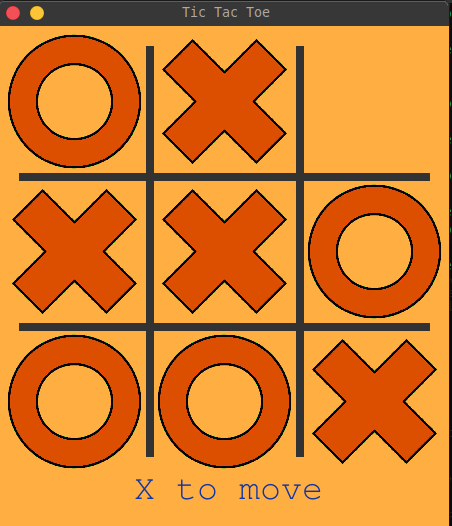Draw match:Horizontal pattern win:Vertical pattern win: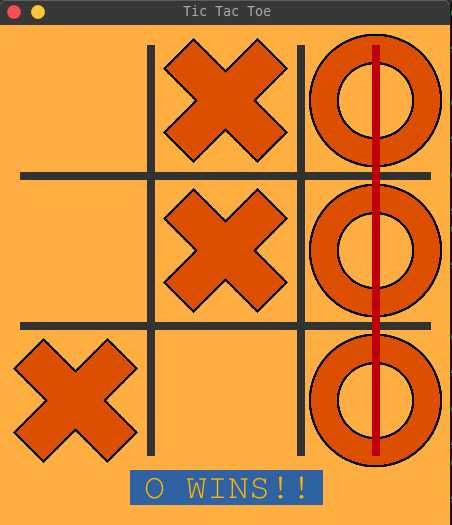Left diagonal pattern win:Right diagonal pattern win:## Conclusion

In conclusion, the game code provided is a Python implementation of the classic Tic-Tac-Toe game using the Pygame library for the graphical user interface. The game allows two players to take turns placing their marks on a 3x3 grid, with the objective of getting three of their marks in a row, either horizontally, vertically, or diagonally.

The code provides a range of functionalities including handling player input, drawing the game board and game pieces, checking for a win or a draw, and displaying appropriate messages and strike lines on the screen.

Overall, this game code is a great starting point for anyone interested in developing simple games using Python and Pygame and can be further extended with additional features and enhancements.

Here are some related games built with Python:

Get the complete code here.

Happy coding ♥

Want to code smarter? Our Python Code Assistant is waiting to help you. Try it now!

Sharing is caring!

### Comment panel

Got a coding query or need some guidance before you comment? Check out our Python Code Assistant for expert advice and handy tips. It's like having a coding tutor right in your fingertips!